Understanding Elementary Shapes (Mathematics) Class 6 - NCERT Questions
Q 1.

What is the disadvantage in comparing line segments by mere observation?

SOLUTION:

There may be chance of error due to improper viewing.

Q 2.

Why is it better to use a divider than a ruler, while measuring the length of a line segment?

SOLUTION:

It is better to use a divider than a ruler, because the thickness of the ruler may cause difficulties in reading of the length of a line segment. However, a divider gives accurate measurement.

Q 3.

Draw any line segment, say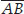. Take any point C lying in between A and B. Measure the lengths of AB, BC and AC. Is AB = AC + CB?
[Note : If A, B, C are any three points on a line such that AC + CB = AB, then we can be sure that C lies between A and B.]

SOLUTION: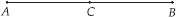AB = 4 cm, AC = 2 cm, CB = 2 cm
Hence, AC + CB = 2 cm + 2 cm = 4 cm = AB
Yes, AB = AC + CB.

Q 4.

If A, B, C are three points on a line such that AB = 5 cm, BC = 3 cm and AC = 8 cm, which one of them lies between the other two?

SOLUTION:

Since,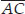is the longest line segment. Thus B is the point lying between A and C.

Q 5.

Verify, whether D is the mid point of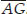.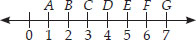SOLUTION: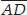= 3 units,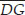= 3 units,=Thus, D is the mid-point of.

Q 6.

If B is the mid point ofand C is the mid point of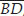, where A, B, C, D lie on a straight line, say why AB = CD?

SOLUTION:

B is the mid point of.
AB = BC ...(I)
And C is the mid point of.
BC = CD ...(ii)
From (I) and (ii), we get
AB = CD

Q 7.

Draw five triangles and measure their sides. Check in each case, if the sum of the lengths of any two sides is always less than the third side.

SOLUTION:

Sum of the lengths of any two sides of a triangle can never be less than length of the third side.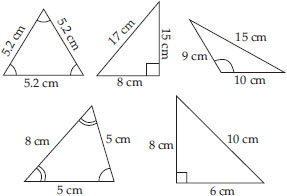Q 8.

What fraction of a clockwise revolution does the hour hand of a clock turn through, when it goes from
(A) 3 to 9
(B) 4 to 7
(C) 7 to 10
(D) 12 to 9
(E) 1 to 10
(F) 6 to 3

SOLUTION:

(A)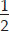or two right angles
(B)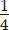or one right angle
(C)or one right angle
(D)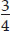or three right angles
(E)or three right angles
(F)or three right angles

Q 9.

Where will the hand of a clock stop if it
(A) starts at 12 and makesof a revolution, clockwise?
(B) starts at 2 and makesof a revolution, clockwise?
(C) starts at 5 and makesof a revolution, clockwise?
(D) starts at 5 and makesof a revolution, clockwise?

SOLUTION:

(A) At 6 (B) At 8 (C) At 8 (D) At 2

Q 10.

Which direction will you face if you start facing
(A) east and makeof a revolution clockwise?
(B) east and make 1of a revolution
clockwise?
(C) west and makeof a revolution anti-clockwise?
(D) south and make one full revolution?
(Should we specify clockwise or anti-clockwise for this last question? Why not?)

SOLUTION:

(A) West
(B) West
(C) North
(D) South
No, it is not necessary to specify clockwise or anti-clockwise because whether we turn clockwise or anti-clockwise, one full revolution will bring us back to the original position.

Q 11.

What part of a revolution have you turned through if you stand facing
(A) east and turn clockwise to face north?
(B) south and turn clockwise to face east?
(C) west and turn clockwise to face east?

SOLUTION:

(A)(B)(C)Q 12.

Find the number of right angles turned through by the hour hand of a clock when it goes from
(A) 3 to 6
(B) 2 to 8
(C) 5 to 11
(D) 10 to 1
(E) 12 to 9
(F) 12 to 6

SOLUTION:

(A) One right angle
(B) Two right angles
(C) Two right angles
(D) One right angle
(E) Three right angles
(F) Two right angles

Q 13.

How many right angles do you make if you start facing
(A) south and turn clockwise to west?
(B) north and turn anti-clockwise to east?
(C) west and turn to west?
(D) south and turn to north?

SOLUTION:

(A) One right angle
(B) Three right angles
(C) Four right angles
(D) Two right angles

Q 14.

Where will the hour hand of a clock stop if it starts
(A) from 6 and turns through 1 right angle?
(B) from 8 and turns through 2 right angles?
(C) from 10 and turns through 3 right angles?
(D) from 7 and turns through 2 straight angles?

SOLUTION:

(A) At 9 (B) At 2 (C) At 7 (D) At 7

Q 15.

Match the following :
 (I) Straight angle of a revolution (A) Less than one-fourth of a revolution (ii) Right angle (B) More than half a revolution (iii) Acute angle (C) Half of a revolution (iv) Obtuse angle (D) One-fourth of a revolution (v) Reflex angle (E) Betweenandof a revolution (F) One complete revolution

SOLUTION:

(I) → (C);
(ii) → (D);
(iii) → (A);
(iv) → (E);
(v) → (B)

Q 16.

Classify each one of the following angles as right, straight, acute, obtuse or reflex :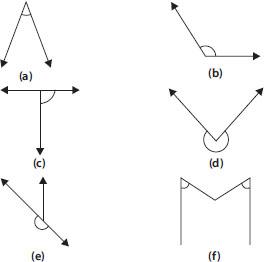SOLUTION:

(A) Acute angle
(B) Obtuse angle
(C) Right angle
(D) Reflex angle
(E) Straight angle
(F) Acute angle.

Q 17.

What is the measure of (I) a right angle? (ii) a straight angle?

SOLUTION:

(I) 90°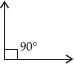(ii) 180°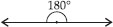Q 18.

Say True or False :
(A) The measure of an acute angle < 90°.
(B) The measure of an obtuse angle < 90°.
(C) The measure of a reflex angle > 180°.
(D) The measure of one complete revolution = 360°.
(E) If mA = 53° and mB = 35°, then
mA > mB.

SOLUTION:

(A) True
(B) False : Since, measure of an obtuse angle is greater than 90° and less than 180°.
(C) True (D) True (E) True

Q 19.

Write down the measures of
(A) some acute angles.
(B) some obtuse angles.
(give at least two examples of each).

SOLUTION:

(A) Measures of 2 acute angles are 35°, 20°
(B) Measures of 2 obtuse angles are 110°, 135°

Q 20.

Measure the angles given below using the Protractor and write down the measure.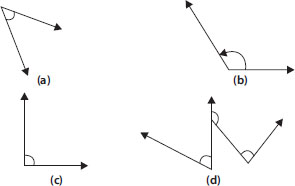SOLUTION:

(D) Near about 60°, 120°, 90°
Note:Students can measure the angles exactly with the help of protractor.

Q 21.

Which angle has a large measure?
First estimate and then measure.
Measure of Angle A =
Measure of Angle B =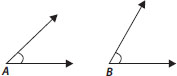SOLUTION:

By estimating, we observe that ∠B has a large measure.
Note: Students can measure the angles exactly with the help of protractor.

Q 22.

From these two angles which has larger measure? Estimate and then confirm by measuring them.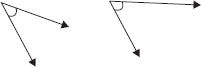SOLUTION:

By estimating, we observe that second angle has larger measure.
First angle is near about 30° and second angle is near about 70°.
Note: Students can measure the angles exactly with the help of protractor.

Q 23.

Fill in the blanks with acute, obtuse, right or straight :
(A) An angle whose measure is less than that of a right angle is______.
(B) An angle whose measure is greater than that of a right angle is ______.
(C) An angle whose measure is the sum of the measures of two right angles is _____.
(D) When the sum of the measures of two angles is that of a right angle, then each one of them is ______.
(E) When the sum of the measures of two angles is that of a straight angle and if one of them is acute then the other should be _______.

SOLUTION:

(A) Acute
(B) Obtuse
(C) Straight
(D) Acute
(E) an Obtuse angle

Q 24.

Find the measure of the angle shown in each figure. (First estimate with your eyes and then find the actual measure with a protractor).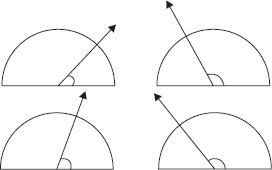SOLUTION:

(I) By estimating with our eyes, we came to know that the measure of angle is 30°.
(ii) By estimating with our eyes, we came to know that the measure of angle is 120°.
(iii) By estimating with our eyes, we came to know that the measure of angle is 60°.
(iv) By estimating with our eyes, we came to know that the measure of angle is 150°.
Note: Students can measure the angles exactly with the help of protractor.

Q 25.

Find the angle measure between the hands of the clock in each figure :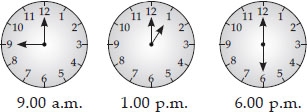SOLUTION:

90° (Right angle), 30° (Acute angle),
180° (Straight angle)

Q 26.

Investigate
In the given figure, the angle measures 30°. Look at the same figure through a magnifying glass. Does the angle becomes larger? Does the size of the angle change?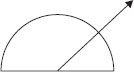SOLUTION:

No, the measure of angle will remain the same.

Q 27.

Measure and classify each angle :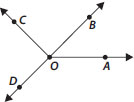Angle Measure Type ∠AOB ∠AOC ∠BOC ∠DOC ∠DOA ∠DOB

SOLUTION:

 Angle Measure Type ∠AOB near about 40° Acute ∠AOC near about 130° Obtuse ∠BOC 90° Right ∠DOC 90° Right ∠DOA near about 140° Obtuse ∠DOB 180° Straight

Note: Students can measure the angles exactly with the help of protractor.

Q 28.

Which of the following are models for perpendicular lines :
(A) The adjacent edges of a table top.
(B) The lines of a railway track.
(C) The line segments forming the letter 'L'.
(D) The letter V.

SOLUTION:

(A) Perpendicular
(B) Not perpendicular
(C) Perpendicular
(D) Not perpendicular

Q 29.

Let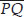be the perpendicular to the line segment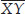. Letandintersect in the point A. What is the measure of ∠PAY?

SOLUTION:

PAY = 90°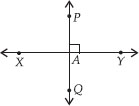Q 30.

There are two set-squares in your box. What are the measures of the angles that are formed at their corners? Do they have any angle measure that is common?

SOLUTION:

One set-square has angles 45°, 90°, 45° and other set-square has angles 60°, 90°, 30°. Yes, they have angle measure 90° as common.

Q 31.

Study the diagram. The line l is perpendicular to line m.
(A) Is CE = EG?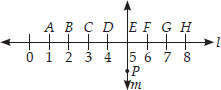(B) Does PE bisect CG?
(C) Identify any two line segments for which PE is the perpendicular bisector.
(D) Are these true?
(I) AC > FG
(ii) CD = GH
(iii) BC < EH.

SOLUTION:

(A) Yes, both measure 2 units.
(B) Yes, because CE = EG
(C)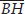and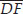are two line segments for which PE is the perpendicular bisector.
(D) (I) True
Since, AC = 2 units and FG = 1 unit.
AC > FG
(ii) True
Since, CD = GH = 1 unit
(iii) True
Since, BC = 1 unit, EH = 3 units.
BC < EH.

Q 32.

Name the types of following triangles :
(A) Triangle with lengths of sides 7 cm, 8 cm and 9 cm.
(B) ΔABC with AB = 8.7 cm, AC = 7 cm and
BC = 6 cm.
(C) ΔPQR such that PQ = QR = PR = 5 cm.
(D) ΔDEF with mD = 90°
(E) ΔXYZ with mY = 90° and XY = YZ.
(F) ΔLMN with mL = 30°, mM = 70° and mN= 80°.

SOLUTION:

(A) Scalene triangle
(B) Scalene triangle
(C) Equilateral triangle
(D) Right-angled triangle
(E) Isosceles right-angled triangle
(F) Acute-angled triangle

Q 33.

Match the following :
Measures of Triangle Type of Triangle
 (I) 3 sides of equal length (A) Scalene (ii) 2 sides of equal length (B) Isosceles right angled (iii) All sides are of different length (C) Obtuse angled (iv) 3 acute angles (D) Right angled (v) 1 right angle (E) Equilateral (vi) 1 obtuse angle (F) Acute angled (vii) 1 right angle with two sides of equal length (G) Isosceles

SOLUTION:

(I) → (E); (ii) → (G); (iii) → (A); (iv) → (F);
(v) → (D); (vi) → (C); (vii) → (B)

Q 34.

Name each of the following triangles in two different ways: (you may judge the nature of the angle by observation)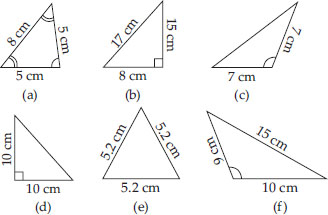SOLUTION:

(A) Acute angled triangle and Isosceles triangle
(B) Right-angled triangle and Scalene triangle
(C) Obtuse-angled triangle and Isosceles triangle
(D) Right-angled triangle and Isosceles triangle
(E) Acute angled triangle and Equilateral triangle
(F) Obtuse-angled triangle and Scalene triangle

Q 35.

Try to construct triangles using match sticks. Some are shown here.
Can you make a triangle with
(A) 3 matchsticks?
(B) 4 matchsticks?
(C) 5 matchsticks?
(D) 6 matchsticks?
(Remember you have to use all the available matchsticks in each case)
Name the type of triangle in each case.
If you cannot make a triangle, think of reasons for it.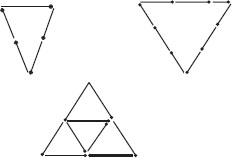SOLUTION:

(A) Yes, it is possible to make a triangle with 3 matchsticks because sum of lengths of two sides is greater than the length of third side.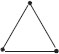(B) No, it is not possible to make a triangle with 4 matchsticks because sum of lengths of two sides is equal to the length of third side.
(C) Yes, it is possible to make a triangle with 5 matchsticks because sum of lengths of two sides is greater than the length of third side.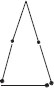(D) Yes, it is possible to make a triangle with the help of 6 matchsticks because sum of lengths of two sides is greater than the length of third side.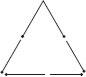Q 36.

Say True or False :
(A) Each angle of a rectangle is a right angle.
(B) The opposite sides of a rectangle are equal in length.
(C) The diagonals of a square are perpendicular to one another.
(D) All the sides of a rhombus are of equal length.
(E) All the sides of a parallelogram are of equal length.
(F) The opposite sides of a trapezium are parallel.

SOLUTION:

(A) True
(B) True
(C) True
(D) True
(E) False : Since, opposite sides of a parallelogram are of equal length.
(F) False : Since, only one pair of opposite sides of a trapezium is parallel.

Q 37.

Give reasons for the following :
(A) A square can be thought of as a special rectangle.
(B) A rectangle can be thought of as a special parallelogram.
(C) A square can be thought of as a special rhombus.
(D) Squares, rectangles, parallelograms are all quadrilaterals.
(E) Square is also a parallelogram.

SOLUTION:

(A) A rectangle with all sides equal becomes a square.
(B) A parallelogram with each angle a right angle becomes a rectangle.
(C) A rhombus with each angle a right angle becomes a square.
(D) All these are four-sided polygons made of line segments.
(E) The opposite sides of a square are parallel, so it is parallelogram.

Q 38.

A figure is said to be regular if its sides are equal in length and angles are equal in measure. Can you identify the regular quadrilateral?

SOLUTION:

A square is a regular quadrilateral.

Q 39.

Examine whether the following are polygons. If any one among them is not, say why?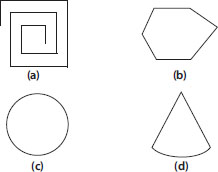SOLUTION:

(A) As it is not a closed figure, therefore, it is not a polygon.
(B) It is a polygon.
(C) It is not a polygon because it is not made by line segments.
(D) It is not a polygon because it is not made by only line segments and also it has curved line.

Q 40.

Name each polygon.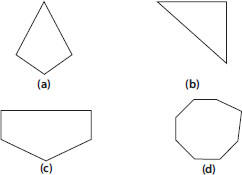Make two more examples of each of these.

SOLUTION:

(B) Triangle
(C) Pentagon
(D) Octagon
Two more examples of each :
(A)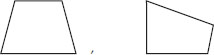(B)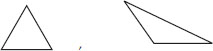(C)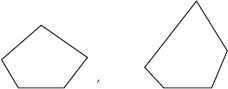(D)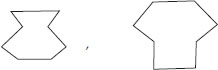Q 41.

Draw a rough sketch of a regular hexagon. Connecting any three of its vertices, draw a triangle. Identify the type of the triangle you have drawn.

SOLUTION: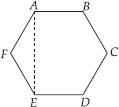ABCDEF is a regular hexagon and ΔAEF is a triangle formed by joining AE. Hence, ΔAEF is an isosceles triangle.

Q 42.

Draw a rough sketch of a regular octagon. (Use squared paper if you wish). Draw a rectangle by joining exactly four of the vertices of the octagon.

SOLUTION: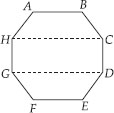ABCDEFGH is a regular octagon and CDGH is a rectangle formed by joining C and H; D and G.

Q 43.

A diagonal is a line segment that joins any two vertices of the polygon and is not a side of the polygon. Draw a rough sketch of a pentagon and draw its diagonals.

SOLUTION: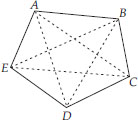ABCDE is the required pentagon and its diagonals are AD, AC, BE, BD and CE.

Q 44.

Match the following :
 (A) Cone (I)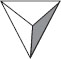(B) Sphere (ii)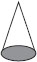(C) Cylinder (iii)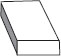(D) Cuboid (iv)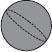(E) Pyramid (v)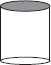Give two new examples of each shape.

SOLUTION:

(A) → (ii);
(B) → (iv);
(C) → (v);
(D) → (iii);
(E) → (I)
Two examples of cone : Ice-cream, Birthday cap
Two examples of sphere : Ball, Rasgulla
Two examples of Cylinder : Pipe, Can
Two examples of Cuboid : Box, Brick
Two examples of a Pyramid : Roof of the house, Cheese grater

Q 45.

What shape is